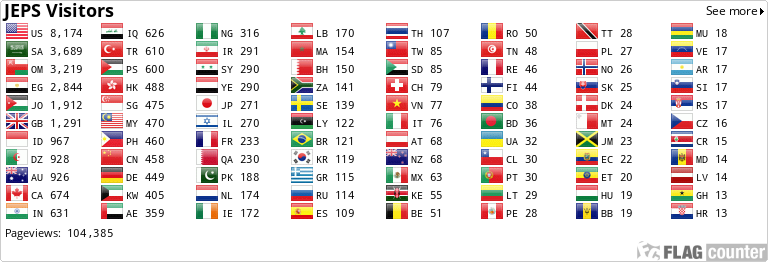### Effect of the Use of the Generative Learning Model on Basic-Stage Students’ Mathematical Problem Solving and Motivation toward Learning Mathematics

#### Abstract

The present study aimed at investigating the effect of the use of the Generative Learning Model on basic-stage students’ mathematical problem solving and motivation toward the learning of mathematics. Two instruments were developed and used in this study: Mathematical Problem Solving Test, which consisted of 15 items, and the Motivation toward the Learning of Mathematics scale comprising 20 items was also used. Validity and reliability of the two instruments were established. The subjects of this study were 77 eighthgrade female students, selected from a basic government school in Nablus. Subjects were divided into two groups: an experimental group 41 students, which was taught according to the generative learning model, and a control one 36 students, which was taught according to the normal way. There were statistically significant differences between the mean scores of the two groups on the mathematical problem solving and motivation toward the learning of mathematics in favor of the experimental group. A number of recommendations were made in light of the findings.

#### Keywords

Generative Learning Model, Mathematical Problem Solving, Motivation toward the Learning of Mathematics, Teaching and Learning Math, Basic-Stage Students.

#### Full Text:

PDF

DOI: http://dx.doi.org/10.24200/jeps.vol6iss2pp1-16

### Refbacks

• There are currently no refbacks.